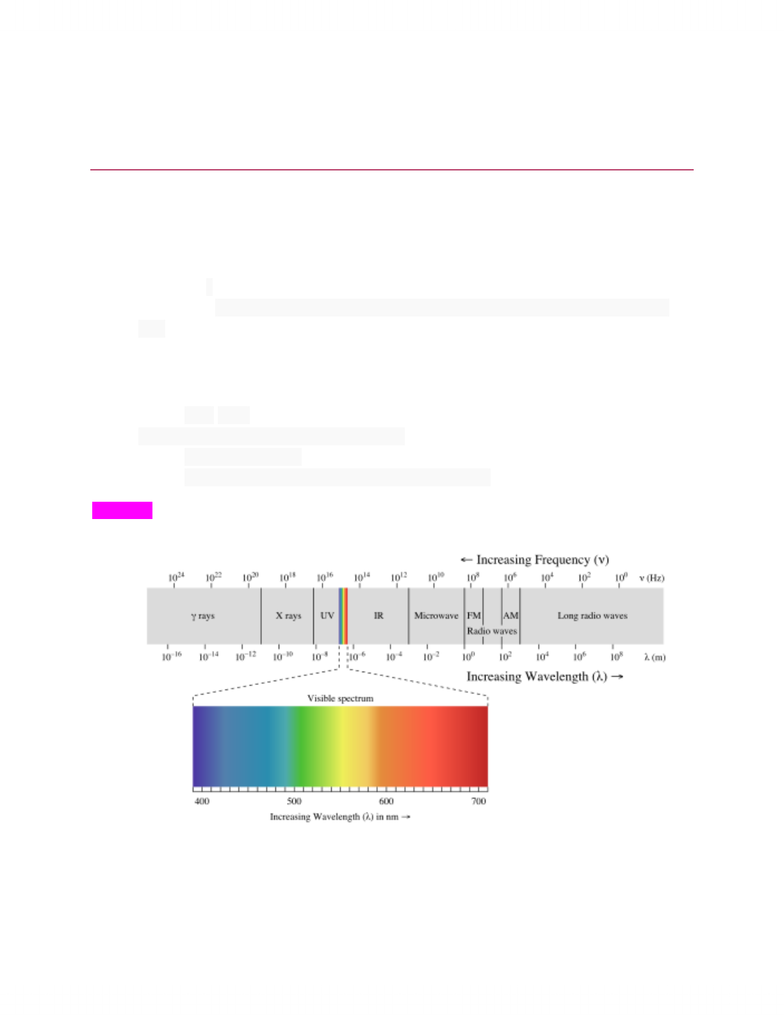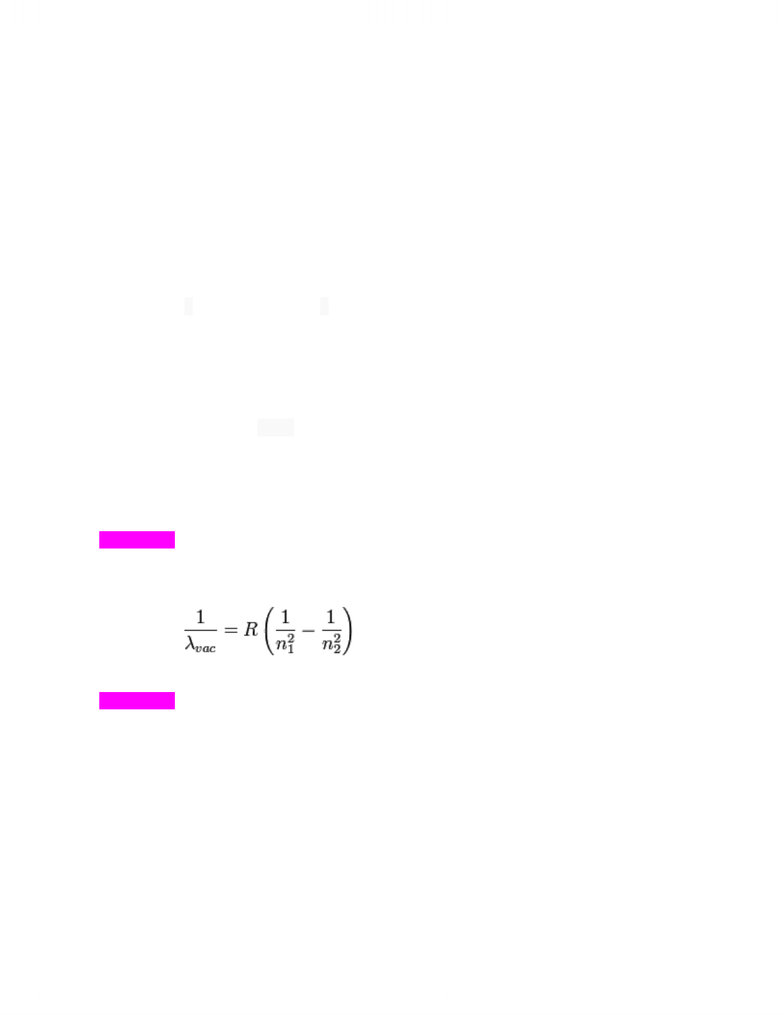Class Notes (1,100,000)
CA (650,000)
U of A (10,000)
CHEM (1,000)
CHEM101 (200)
Lecture

# CHEM101 Lecture Notes - Angular Momentum, Black Body, Rydberg Formula

This preview shows pages 1-2. to view the full 6 pages of the document.Silberberg: 5th ed.
7: Quantum Theory & Atomic Structure
7.1 The Nature of Light
Wave Nature of Light
Frequency (ν) cycles/ second (aka “hertz” = Hz)
Wavelength (λ) distance from one crest/trough to the next crest/trough (in meters/nm,
etc.)
*frequency + wavelength inversely proportional
Speed of light (c) 2.9979E8 m/s
o (ν) x (λ) = c
Amplitude height of crest/ depth of trough
o Related to intensity
o Lower amplitude = dimmer/ higher amp. = brighter
Know how: to convert frequency <-> wavelength
Refraction change of speed as light wave travels through different mediums
Diffraction waves bending around an object
o Ex: light wave passing through slits = constructive interference (in phase/bright spot),
destructive interference (out of phase/ dark spot)

Only pages 1-2 are available for preview. Some parts have been intentionally blurred.Particle Nature of Light
1900 Planck’s constant
o Proposed that blackbodies could only emit/absorb certain quantities of energy
E =nhv
E = energy
v = frequency
h = Planck’s constant = 6.626E-34 J/s
Energy gained/lost in “packets” (quantum)
o Δ Eatom = Eemitted/absorbed = Δ nhv
Photoelectric effect not explained by wave model
o Presence of threshold frequency: minimum frequency needed for a current
o Absence of a time lag: current flows immediately once minimum threshold frequency
met
Sometime after Planck Einstein’s photon theory
o Ephoton = hv = ΔEatom
o Explains threshold frequency: photon of minim. Energy needs to be absorbed in order to
free an electron
o Explains time lag: brighter light = more photons = faster current once absorbed one
photon of enough energy
*Know how to: calculate energy of radiation from its wavelengths (and vice versa)
7.2 Atomic Spectra
Rydberg equation predicts position and wavelength of any line in a given series
o
o R = Rydberg constant = 1.096776E7 m/s
*Know how to: use Rydberg equation
Bohr Model of the Hydrogen Atom
Explains that energy within an atom only exists in states/ certain discrete levels
Lower n value = smaller radius of orbit = lower energy level
o n=1 -> ground state
o n=2,3,etc. -> excited state (n=2 = first excited state)
o n= -> ionized